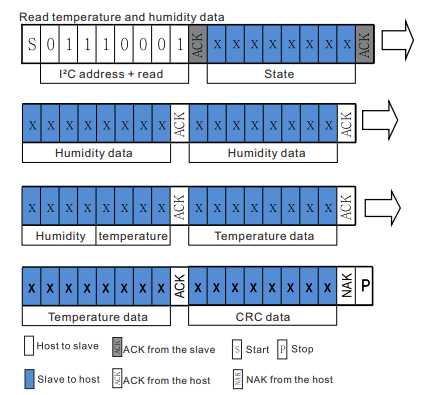# B4R Question20-bit uLong bit shifts

#### peacemaker

##### Expert
Longtime User
HI, All

AHT21 sensor (humidity and temperature) returns 5 bytes of data (among 7):Some C-code of the lib to convert is:

B4X:
``````  uint32_t humidity   = _rawData;                          //20-bit raw humidity data
humidity <<= 8;
humidity  |= _rawData;
humidity <<= 4;
humidity  |= _rawData >> 4;

uint32_t temperature   = _rawData & 0x0F;                //20-bit raw temperature data
temperature <<= 8;
temperature  |= _rawData;
temperature <<= 8;
temperature  |= _rawData;``````

How to make this in B4R ?

Bit.operations are only for 16-bit UInt, how to merge these 2.5 bytes to ULong 32-bit variables ?

Last edited:

#### peacemaker

##### Expert
Longtime User
Solved, but not by bit shifts

B4X:
``````        Dim h0 As Byte = raf.ReadByte(1)
Dim h1 As Byte = raf.ReadByte(2)
Dim h2 As Byte = raf.ReadByte(3)
h2 = Bit.ShiftRight(h2, 4)
Dim h As ULong = h0 * 0x1000 + h1 * 0x10 + h2
If h > 0x100000 Then
h = 0x100000
End If
Humidity = h / 0x100000 * 100    '%

Dim t0 As Byte = raf.ReadByte(3)
Dim t1 As Byte = raf.ReadByte(4)
Dim t2 As Byte = raf.ReadByte(5)
t0 = Bit.And(t0, 0x0F)
Dim t As ULong = t0 * 0x10000 + t1 * 0x100 + t2
Tmp = t / 0x100000 * 200 - 50

Log("h = ", h, "; t = ", t)
Log("Humidity = ", Humidity, "; Tmp = ", Tmp)``````

B4X:
``````h = 221095; t = 401153
Humidity = 21.0853; Tmp = 26.5139``````

Last edited:
•emexes

#### emexes

##### Expert
This constraint shouldn't be needed, since h will never exceed 0xFFFFF (since h0 and h1 are max 0xFF and h2 is max 0x0F):
B4X:
``````Dim h As ULong = h0 * 0x1000 + h1 * 0x10 + h2
If h > 0x100000 Then
Humidity = 0x100000
End If``````

Also, why assign to Humidity when it is immediately overwritten anyway? :
B4X:
``````If h > 0x100000 Then
Humidity = 0x100000
End If
Humidity = h / 0x100000 * 100    '%``````

Also, Humidity can never be exactly 100.0% since h will never be 0x100000 (never exceed 0xFFFFF). Not that it matters much, we're only talking about one part in a million, which I assume will be buried by sensor noise anyway. But for some use cases, exact correctness counts.edit: now that I've looked at the datasheet... your conversion is per their gospel, so no worriesbut if this is a uni project then it might still get you bonus points for the observation about the intrinsic limit on the Humidity readingLast edited:
•peacemaker

#### peacemaker

##### Expert
Longtime User
Thanks for analysis, fixed the IF. I was just trying to overwrite the lib code as is...
But anyway my solution is a workaround, actually it's interesting how to do with the bit shifts... It looks like much harder in B4R.

Last edited:

#### emexes

##### Expert
it's interesting how to do with the bit shifts... It looks like much harder in B4R.

Yeah, the 16-bit limit on shifts is a bummer but if ByteConverter works then you can roll your own ULong versions eg (not tested) :

B4X:
``````Sub BitShiftRight(UL as ULong, N as Byte) As ULong

If N = 0 Then    'probably a premature optimization
Return UL
Else If N >= 32 Then
Return 0
End If

Dim bc as ByteConverter

Dim B() As Byte = bc.ULongsToBytes(Array As ULong(UL))

Do While N >= 8
B(0) = B(1)
B(1) = B(2)
B(2) = B(3)
B(3) = 0
N = N - 8
Loop

If N <> 0 Then
'        high N bits              low 8 - N bits
'==========================  =======================
B(0) = Bit.Or(Bit.ShiftLeft(B(1), 8 - N), Bit.ShiftRight(B(0), N))
B(1) = Bit.Or(Bit.ShiftLeft(B(2), 8 - N), Bit.ShiftRight(B(1), N))
B(2) = Bit.Or(Bit.ShiftLeft(B(3), 8 - N), Bit.ShiftRight(B(2), N))
B(3) =                                    Bit.ShiftRight(B(3), N)
End If

Return bc.ULongsFromBytes(B)(0)

End Sub``````

heck that was more complicated than I first thoughthappily, left shifts are just multiplies eg by 0x100 to shift left 8 bits, or more generally, 2^n to shift left n bits

edit: I can see now that BitLeftShift should have chunked the ULong down to 16-bit UInts rather than 8-bit Bytes...Last edited:
•peacemaker

Replies
0
Views
2K
Replies
16
Views
3K